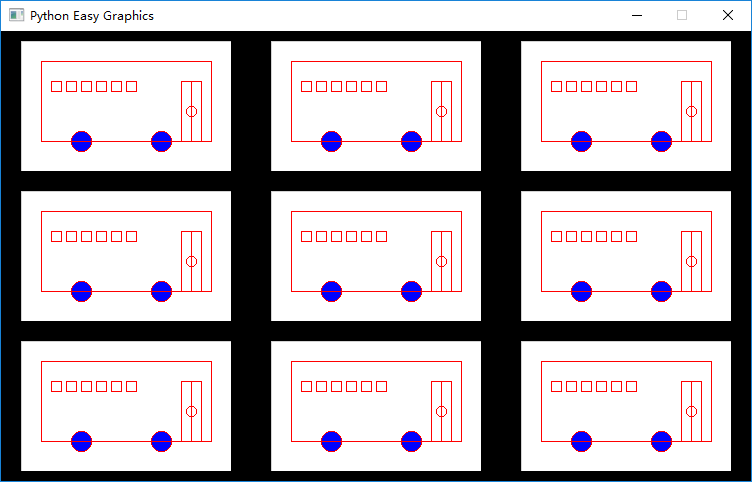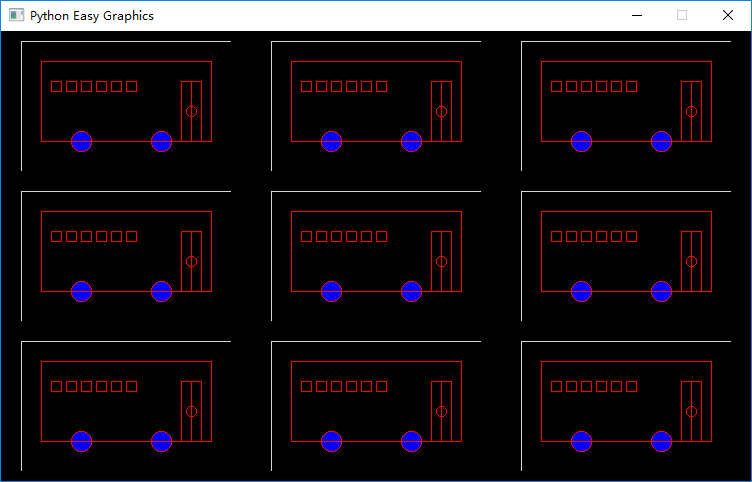# 图片处理¶

## 创建和绘制图片¶

1. 面向对象方式：使用图片对象的成员方法。create_image()创建的是一个图片对象。我们可以直接使用它的成员方法。
```img=create_image(800,600)
img.draw_circle(100,100,50)
```
1. 传统方式：使用前面章节中的绘图函数，同时指明在哪个图片上绘制（缺省在绘图窗口中绘制）
```img=create_image(400,300)
draw_circle(100,100,50,img)
```

## 绘图窗口和绘制目标（target）¶

```img=create_image(400,300)
set_target(img)
draw_circle(100,100,50)
```

## 保存图片¶

1. save_image()缺省保存“绘制目标”图片；
2. 缺省的“绘制目标”是绘图窗口。

```from easygraphics import *
import draw_bus

def main():
init_graph(600,400)
draw_bus.draw_bus()
save_image("bus_screen.png")
pause()
close_graph()

easy_run(main)
```

## 复制图片¶

1. 创建一个图片对象，保存到变量”img”中。
2. 将“绘制目标”设为”img”。
3. 在“绘制目标”（”img”图片）上画一辆公共汽车
4. 将“绘制目标”设为绘图窗口
5. 将”img”中的内容复制到绘图窗口的不同位置
```from easygraphics import *
import draw_bus

def main():
init_graph(750, 450)
img = create_image(210, 130)
set_target(img)  # set target to img
draw_bus.draw_bus()
set_target()  # set target back to the graphics window
set_background_color("black")
for i in range(0, 9):
x = i % 3 * 250
y = i // 3 * 150
draw_image(x + 20, y + 10, img)

pause()
img.close()
close_graph()

easy_run(main)
```## 图片透明和合成¶

put_image()从源图片复制一个矩形区域到目标图片上。如果你需要复制一个外形轮廓不是矩形的图像，可以先将其画在一个背景完全透明的图片上，然后再复制。

```from easygraphics import *
import draw_bus

def main():
init_graph(750, 450)
img = create_image(210, 130)
set_background_color(Color.TRANSPARENT, img)  # set img's background to transparency
set_target(img)  # set target to img
draw_bus.draw_bus()
set_target()  # set target back to the graphics window
set_background_color("black")
for i in range(0, 9):
x = i % 3 * 250
y = i // 3 * 150
draw_image(x + 20, y + 10, img)

pause()
img.close()
close_graph()

easy_run(main)
```## 复制图片时忽略背景¶

```from easygraphics import *
import draw_bus

def main():
init_graph(750, 450)
img = create_image(210, 130)
set_target(img)  # set target to img
draw_bus.draw_bus()
set_target()  # set target back to the graphics window
set_background_color("black")
for i in range(0, 9):
x = i % 3 * 250
y = i // 3 * 150
draw_image(x + 20, y + 10, img, with_background=False)

pause()
img.close()
close_graph()

easy_run(main)
```## 带几何变换的图片复制¶

```from easygraphics import *
import draw_bus

def main():
init_graph(750, 450)
img = create_image(210, 130)
set_target(img)  # set target to img
draw_bus.draw_bus()
set_target()  # set target back to the graphics window
set_background_color("black")
for i in range(0, 9):
x = i % 3 * 250 + 20
y = i // 3 * 150 + 10
save_settings()
# transforms
translate(x, y)
translate(105, 65)
rotate(45)
translate(-105, -65)

draw_image(0, 0, img, with_background=False)
restore_settings()
pause()
img.close()
close_graph()

easy_run(main)
```## 读入图片文件¶

```from easygraphics import *

def main():
init_graph(800, 600)
draw_image((get_width() - img.get_width()) // 2,
(get_height() - img.get_height()) // 2, img)
pause()
img.close()
close_graph()

easy_run(main)
```

```from easygraphics import *
import draw_bus

def main():